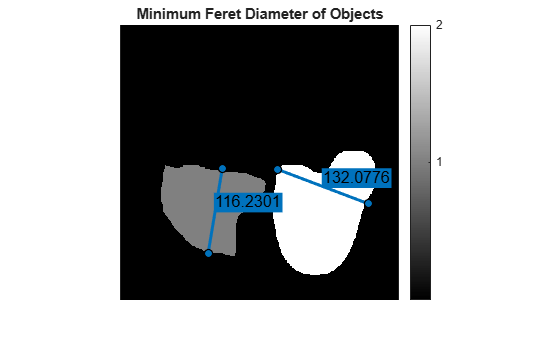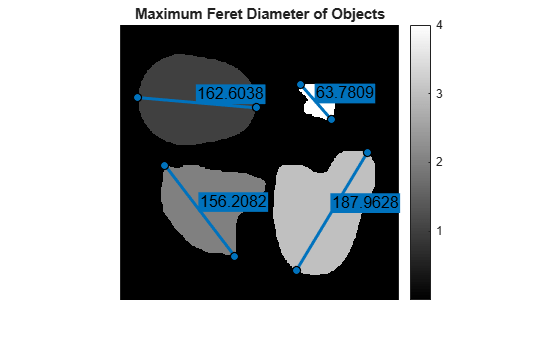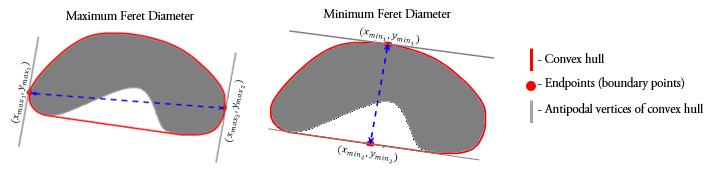# bwferet

Measure Feret properties

Since R2019a

## Syntax

``out = bwferet(BW,properties)``
``out = bwferet(CC,properties)``
``out = bwferet(L,properties)``
``out = bwferet(input)``
``[out,LM] = bwferet(___)``

## Description

example

````out = bwferet(BW,properties)` measures the Feret properties of objects in an image and returns the measurements in a table. The input `properties` specifies the Feret properties to be measured for each object in input binary image `BW`. The measured Feret properties include the minimum and maximum Feret diameters, Feret angles, and endpoint coordinates of Feret diameters.```

example

````out = bwferet(CC,properties)` measures the Feret properties for each connected component in structure `CC`.```
````out = bwferet(L,properties)` measures the Feret properties for each object in the input label matrix `L`.```
````out = bwferet(input)` measures the maximum Feret diameter, its relative angle, and coordinate values measured from the `input`. The function returns the measurements in a table. The `input` can be binary image `BW`, connected component `CC`, or label matrix `L`.```
````[out,LM] = bwferet(___)` also returns a label matrix containing label values that represent the row indices of the table `out`. You can use any of the input arguments from previous syntaxes. Each row entry in `out` corresponds to a labeled region (object) in label matrix `LM`. ```

## Examples

collapse all

Read an image into the workspace.

`I = imread('toyobjects.png');`

Convert the image into a binary image.

`bw = imbinarize(I,'adaptive');`

Extract the first two largest objects from the binary image.

`bw = bwareafilt(bw,2);`

Fill holes in the extracted object regions.

`bw = imfill(bw,'holes');`

Calculate the minimum Feret properties and the label matrix of the extracted objects.

`[out,LM] = bwferet(bw,'MinFeretProperties');`

Get the maximum number of objects in the output label matrix.

`maxLabel = max(LM(:));`

Display the output containing the table of minimum Feret properties.

`out`
```out=2×3 table MinDiameter MinAngle MinCoordinates ___________ ________ ______________ 116.23 99.462 {2x2 double} 132.08 -159.27 {2x2 double} ```

Display the minimum Feret properties of the object with label-value 1 from the output label matrix.

`out.MinDiameter(1)`
```ans = 116.2301 ```
`out.MinAngle(1)`
```ans = 99.4623 ```
`out.MinCoordinates{1}`
```ans = 2×2 120.5000 311.5000 139.6081 196.8514 ```

Display the minimum Feret properties of the object with label-value 2 from the output label matrix.

`out.MinDiameter(2)`
```ans = 132.0776 ```
`out.MinAngle(2)`
```ans = -159.2744 ```
`out.MinCoordinates{2}`
```ans = 2×2 215.5000 197.5000 339.0304 244.2412 ```

Display the output label matrix. Plot the endpoint coordinates and minimum Feret diameter of objects with different label values from the output label matrix.

```h = imshow(LM,[]); axis = h.Parent; for labelvalues = 1:maxLabel xmin = [out.MinCoordinates{labelvalues}(1,1) out.MinCoordinates{labelvalues}(2,1)]; ymin = [out.MinCoordinates{labelvalues}(1,2) out.MinCoordinates{labelvalues}(2,2)]; imdistline(axis,xmin,ymin); end title(axis,'Minimum Feret Diameter of Objects'); colorbar('Ticks',1:maxLabel)```Read an image into the workspace.

`I = imread('toyobjects.png');`

Convert the image into a binary image.

`bw = imbinarize(I,'adaptive');`

Fill holes in the object regions of the input binary image.

`bw = imfill(bw,'holes');`

Use the `bwconncomp` function to generate connected components from the resulting image.

`cc = bwconncomp(bw);`

Measure the maximum Feret properties of the connected components.

`[out,LM] = bwferet(cc,'MaxFeretProperties');`

Get the maximum number of objects in the output label matrix.

`maxLabel = max(LM(:));`

Inspect the table to verify the measured maximum Feret properties.

`out`
```out=4×3 table MaxDiameter MaxAngle MaxCoordinates ___________ ________ ______________ 162.6 -175.06 {2x2 double} 156.21 -127.46 {2x2 double} 187.96 121.07 {2x2 double} 63.781 -131.19 {2x2 double} ```

Display the maximum Feret diameters of objects with different label values from output label matrix.

`out.MaxDiameter(1:maxLabel)`
```ans = 4×1 162.6038 156.2082 187.9628 63.7809 ```

Display the directional angles of the maximum Feret diameters specific to objects with different label values from output label matrix.

`out.MaxAngle(1:maxLabel)`
```ans = 4×1 -175.0608 -127.4568 121.0683 -131.1859 ```

Display the endpoint coordinates of the maximum Feret diameters specific to objects with different label values from output label matrix.

`out.MaxCoordinates{1:maxLabel}`
```ans = 2×2 186.5000 113.5000 24.5000 99.5000 ```
```ans = 2×2 156.5000 315.5000 61.5000 191.5000 ```
```ans = 2×2 337.5000 174.5000 240.5000 335.5000 ```
```ans = 2×2 288.5000 129.5000 246.5000 81.5000 ```

Display the output label matrix. Plot the endpoint coordinates and the maximum Feret diameter of objects with different label values from output label matrix.

```h = imshow(LM,[]); axis = h.Parent; for labelvalues = 1:maxLabel xmax = [out.MaxCoordinates{labelvalues}(1,1) out.MaxCoordinates{labelvalues}(2,1)]; ymax = [out.MaxCoordinates{labelvalues}(1,2) out.MaxCoordinates{labelvalues}(2,2)]; imdistline(axis,xmax,ymax); end title(axis,'Maximum Feret Diameter of Objects'); colorbar('Ticks',1:maxLabel)```## Input Arguments

collapse all

Binary image, specified as a logical or numeric matrix. For numeric input, any nonzero pixels are considered to be `1` (`true`).

Data Types: `single` | `double` | `int8` | `int16` | `int32` | `uint8` | `uint16` | `uint32` | `logical`

Connected components, specified as a structure with the four fields shown in this table.

FieldDescription
`Connectivity`Connectivity of the connected components (objects)
`ImageSize`Size of input binary image
`NumObjects`Number of connected components (objects) in the input binary image
`PixelIdxList`1-by-`NumObjects` cell array, where the kth element is a vector containing the linear indices of the pixels in the kth object

You can use the `bwconncomp` function to generate connected components from a binary image.

Data Types: `struct`

Label matrix of contiguous regions, specified as a matrix of nonnegative integers. The pixels labeled 0 are the background. The pixels labeled 1 make up one object; the pixels labeled 2 make up a second object; and so on. The number of objects represented by `L` is equal to the maximum value of `L`. You can use the `bwlabel` function to generate label matrix from a binary image.

Data Types: `single` | `double` | `int8` | `int16` | `int32` | `uint8` | `uint16` | `uint32` | `logical`

Label for Feret properties, specified as `"MaxFeretProperties"`, `"MinFeretProperties"`, or `"all"`.

Data Types: `char` | `string`

Generic input, specified as one of these values:

• Numeric matrix or logical matrix — When `input` is a binary image, `BW`.

• Structure — When `input` is the connected component, `CC`.

• Matrix of nonnegative integers — When `input` is the label matrix, `L`.

Data Types: `single` | `double` | `int8` | `int16` | `int32` | `uint8` | `uint16` | `uint32` | `logical` | `struct`

## Output Arguments

collapse all

Table of Feret properties, returned as an m-by-n table. m is the number of objects for which the Feret properties are measured. n is 3 or 6, depending on the `properties` input.

• If `properties` is `"MaxFeretProperties"`, then the table `out` is of size m-by-3 with columns `MaxDiameter`, `MaxAngle`, and `MaxCoordinates`.

• If `properties` is `"MinFeretProperties"`, then the table `out` is of size m-by-3 with columns `MinDiameter`, `MinAngle`, and `MinCoordinates`.

• If `properties` is `"all"`, then the table `out` is of size m-by-6 with all columns listed in this table.

Column NameDescription
`MaxDiameter`Maximum Feret diameter of an object, measured as the maximum distance between any two boundary points on the antipodal vertices of the convex hull that encloses that object
`MaxAngle`

Directional angle of the maximum Feret diameter with respect to the horizontal axis of the image. The value, in degrees, is in the range [–180o,180o]

`MaxCoordinates`Endpoint coordinates of the maximum Feret diameter, returned in the form $\left[\begin{array}{l}{x}_{1}\text{\hspace{0.17em}}\text{\hspace{0.17em}}\text{\hspace{0.17em}}\text{\hspace{0.17em}}\text{\hspace{0.17em}}{y}_{1}\\ {x}_{2}\text{\hspace{0.17em}}\text{\hspace{0.17em}}\text{\hspace{0.17em}}\text{\hspace{0.17em}}\text{\hspace{0.17em}}{y}_{2}\end{array}\right]$
`MinDiameter`Minimum Feret diameter of an object, measured as the minimum distance between any two boundary points on the antipodal vertices of the convex hull that encloses that object
`MinAngle`

Directional angle of the minimum Feret diameter with respect to the horizontal axis of the image. The value, in degrees, is in the range [–180o,180o]

`MinCoordinates`Endpoint coordinates of the minimum Feret diameter, returned in the form $\left[\begin{array}{l}{x}_{1}\text{\hspace{0.17em}}\text{\hspace{0.17em}}\text{\hspace{0.17em}}\text{\hspace{0.17em}}\text{\hspace{0.17em}}{y}_{1}\\ {x}_{2}\text{\hspace{0.17em}}\text{\hspace{0.17em}}\text{\hspace{0.17em}}\text{\hspace{0.17em}}\text{\hspace{0.17em}}{y}_{2}\end{array}\right]$

Label matrix of contiguous regions, specified as a matrix of nonnegative integers. The pixels labeled 0 are the background. The pixels labeled 1 make up one object; the pixels labeled 2 make up a second object; and so on. The Feret properties in the kth row entry of `out` correspond to the kth region (object) in `LM` that have the value k. The number of objects represented by `LM` is equal to the maximum value of `LM`.

Note

If the input to `bwferet` is a label matrix, then the output label matrix `LM` is same as the input label matrix.

Data Types: `uint8`

## Algorithms

The Feret properties of an object are measured by using boundary points on the antipodal vertices of the convex hull that encloses that object.Given the endpoint coordinates of the maximum (or minimum) Feret diameter, $\left[\begin{array}{l}{x}_{1}\text{\hspace{0.17em}}\text{\hspace{0.17em}}\text{\hspace{0.17em}}\text{\hspace{0.17em}}\text{\hspace{0.17em}}{y}_{1}\\ {x}_{2}\text{\hspace{0.17em}}\text{\hspace{0.17em}}\text{\hspace{0.17em}}\text{\hspace{0.17em}}\text{\hspace{0.17em}}{y}_{2}\end{array}\right]$, the maximum (or minimum) Feret angle is measured as $angle\text{\hspace{0.17em}}\text{\hspace{0.17em}}=\text{\hspace{0.17em}}\text{\hspace{0.17em}}{\mathrm{tan}}^{-1}\left(\frac{{y}_{2}-{y}_{1}}{{x}_{2}-{x}_{1}}\right)$.

## Version History

Introduced in R2019a

expand all